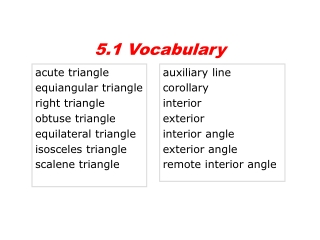DownloadDownload Presentationacute triangle equiangular triangle right triangle obtuse triangle equilateral triangle

# acute triangle equiangular triangle right triangle obtuse triangle equilateral triangle

Download Presentation## acute triangle equiangular triangle right triangle obtuse triangle equilateral triangle

- - - - - - - - - - - - - - - - - - - - - - - - - - - E N D - - - - - - - - - - - - - - - - - - - - - - - - - - -
##### Presentation Transcript

1. 5.1 Vocabulary acute triangle equiangular triangle right triangle obtuse triangle equilateral triangle isosceles triangle scalene triangle auxiliary line corollary interior exterior interior angle exterior angle remote interior angle

2. C A AB, BC, and AC are the sides of ABC. B Recall that a triangle ( ) is a polygon with three sides. Triangles can be classified in two ways: by their angle measures or by their side lengths. A, B, C are the triangle's vertices.

3. Example 1A: Classifying Triangles by Angle Measures Classify BDC by its angle measures. Classify ABD by its angle measures.

4. Example 1B: Classifying Triangles by Side Lengths Classify EHF by its side lengths. Classify EHGby its side lengths.

5. Quiz Classify each triangle by its angles and sides. 1. MNQ 2.NQP 3. MNP 4. Find the side lengths of the triangle.

6. 5 - 1 An auxiliary line is a line that is added to a figure to aid in a proof. An auxiliary line used in the Triangle Sum Theorem

7. 118° Example 2: Application After an accident, the positions of cars are measured by law enforcement to investigate the collision. Use the diagram drawn from the information collected to find mYWZ. Step 1 Find mWXY. mYXZ + mWXY = 180° Lin. Pair Thm. and  Add. Post. 62 + mWXY = 180 Substitute 62 for mYXZ. mWXY = 118° Subtract 62 from both sides.

8. A corollary is a theorem whose proof follows directly from another theorem. Here are two corollaries to the Triangle Sum Theorem. 5-1-a 5-1-b

9. Example 3: Finding Angle Measures in Right Triangles One of the acute angles in a right triangle measures 2x°. What is the measure of the other acute angle? (answer in terms of “x”)

10. The interior is the set of all points inside the figure. The exterior is the set of all points outside the figure. An interior angle is formed by two sides of a triangle. An exterior angle is formed by one side of the triangle and extension of an adjacent side. 4 is an exterior angle. Exterior Interior 3 is an interior angle.

11. Each exterior angle has two remote interior angles. A remote interior angle is an interior angle that is not adjacent to the exterior angle. 4 is an exterior angle. The remote interior angles of 4 are 1 and 2. Exterior Interior 3 is an interior angle.

12. 5-2 Example 3: Applying the Exterior Angle Theorem Find mB.

13. 2 3 Lesson Quiz: Part I 1. The measure of one of the acute angles in a right triangle is 56 °. What is the measure of the other acute angle? 2. Find mABD. 3. Find mN and mP.

14. Lesson Quiz: Part II 4. The diagram is a map showing John's house, Kay's house, and the grocery store. What is the angle the two houses make with the store? 30°